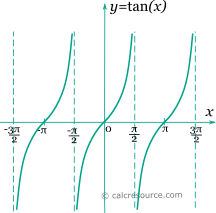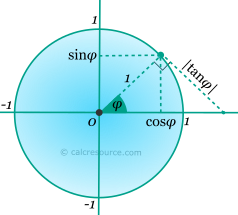Trigonometric tangent calculator

This tool evaluates the tangent of a number: tan(x). Enter the argument x, in radians or degrees, below.

 x = deg rad Result: tan(x) = Also, at same x: sin(x) = cos(x) = cot(x) =Definitions

General

The tangent function, in modern notation written as tan(x), is a trigonometric function. Trigonometric functions are commonly established as functions of angle, in the context of right triangle geometry. This way, the tangent of an angle φ is defined as the ratio of the opposite side of a right triangle containing φ, divided by the adjacent side (see figure):Unfortunately the above definition is limited to a range of angles between 0 and 90°. Extending that range, a convenient definition employs a unit circle (radius equal to 1). Any point of the circle, corresponds to a pair of sine and cosine values, of the angle that is contained by the horizontal positive axis and the radial segment towards that point, as shown in the figure below. The absolute value of tangent is represented by the length of the tangent segment from the circle point towards the horizontal axis. The angle φ is assumed positive in counter-clockwise direction. Angles larger than 90°, as well as negative ones, are possible, if the appropriate sign of the coordinates is respected.The above definitions of tangent function are based on a geometrical construct (right triangle or unit circle) and assume that its argument is an angle. This association to an angle is restrictive, considering the broad use of trigonometric functions in mathematics, physics, engineering etc. Applications may accept any real value as argument, with any imaginable meaning given to it, or no meaning at all. In that case, x should be better measured in radians (). Thus, derivation and integration rules are more conveniently applied.

Series

All trigonometric functions can be defined in an infinite series form. Tangent function can be written as:

The above series converges for . Bndenotes the n-th Bernulli number .

Properties

The tangent function is periodic, with a period equal to π. Therefore:

Tangent is associated with sine and cosine functions with the formula:

The derivative of the tangent function (x in radians) is given by the following expressions:

The integral of the tangent function (x in radians) is given by:

Identities

The following formulas are valid for the tangent function: